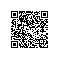# Java核心技术卷I基础知识3.9　大数值

3.9　大数值

API java.math.BigInteger 1.1

BigInteger subtract(BigInteger other)

BigInteger multiply(BigInteger other)

BigInteger divide(BigInteger other)

BigInteger mod(BigInteger other)

int compareTo(BigInteger other)

static BigInteger valueOf(long x)

java.math.BigInteger 1.1

BigDecimal subtract(BigDecimal other)

BigDecimal multiply(BigDecimal other)

BigDecimal divide(BigDecimal other，RoundingMode mode) 5.0

int compareTo(BigDecimal other)

static BigDecimal valueOf(long x)

static BigDecimal valueOf(long x,int scale)